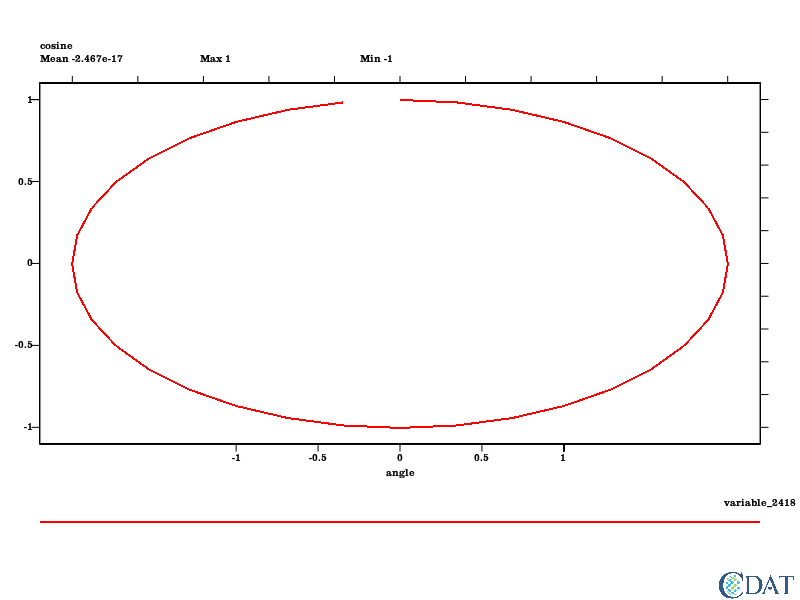# 1D Plots Tutorial¶

This tutorial is for creating 1D lines plot using CDAT's VCS.

The CDAT software was developed by LLNL. This tutorial was written by Charles Doutriaux. This work was performed under the auspices of the U.S. Department of Energy by Lawrence Livermore National Laboratory under Contract DE-AC52-07NA27344.

Download the Jupyter Notebook

## Create VCS canvas¶

Back to Top

In :
import vcs
x = vcs.init(bg=True,geometry=(800,600))


## Prepare input data¶

Back to Top

Let's start with simple sine function

In :
import numpy
angle = numpy.arange(0, 360, 10) # in degrees
s = numpy.sin(angle/180.*numpy.pi) # sin takes radians as input


## Very Basic Plot¶

Back to Top

In its most basic form VCS does not need anything else to plot:

In :
x.plot(s)

Out: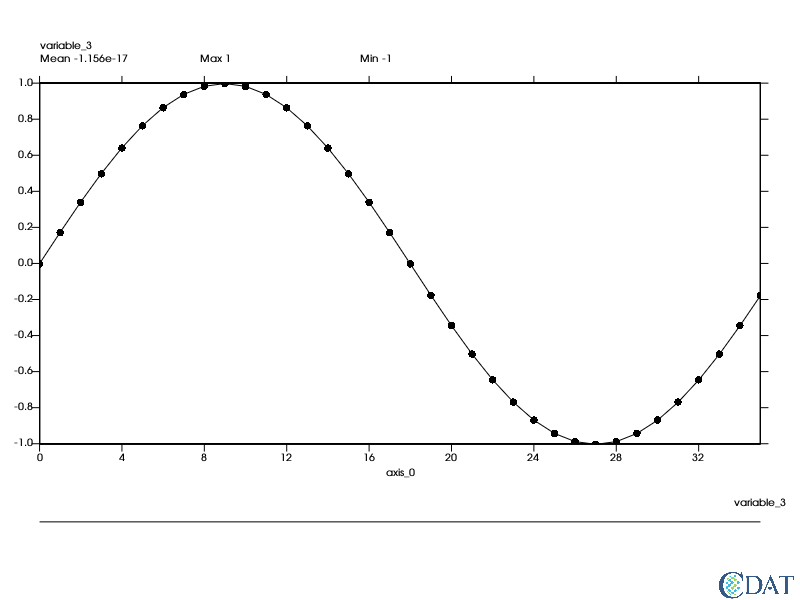## Basic Controls¶

Back to Top

Let's change the color of the line and size aspect and size of the markers.

For this we will need to create a 1D graphic method and alter some of its attributes

The following is list of available markersIn :
line = vcs.create1d()
line.list()  # List what we can change

---------- Yxvsx (GYx) member (attribute) listings ----------
graphics method = G1d
name = __1d_492654641158574
projection = linear
xticlabels1 = *
xticlabels2 = *
xmtics1 =
xmtics2 =
yticlabels1 = *
yticlabels2 = *
ymtics1 =
ymtics2 =
datawc_x1 = 1e+20
datawc_y1 =  1e+20
datawc_x2 =  1e+20
datawc_y2 =  1e+20
datawc_timeunits =  days since 2000
datawc_calendar =  135441
xaxisconvert =  linear
yaxisconvert =  linear
linetype =  solid
linecolor =  [0.0, 0.0, 0.0, 100.0]
linewidth =  1
marker =  dot
markercolor =  [0.0, 0.0, 0.0, 100.0]
markersize =  1
flip =  False

In :
line.linecolor = "red"  # Red color obviously
line.linewidth = 2.  # A bit thicker
line.marker = "circle"  #
line.markersize = 1.25  # A bit bigger
x.clear() # Let's clear the first plot
x.switchfonts("default","Clarendon")
x.plot(s,line)

Out: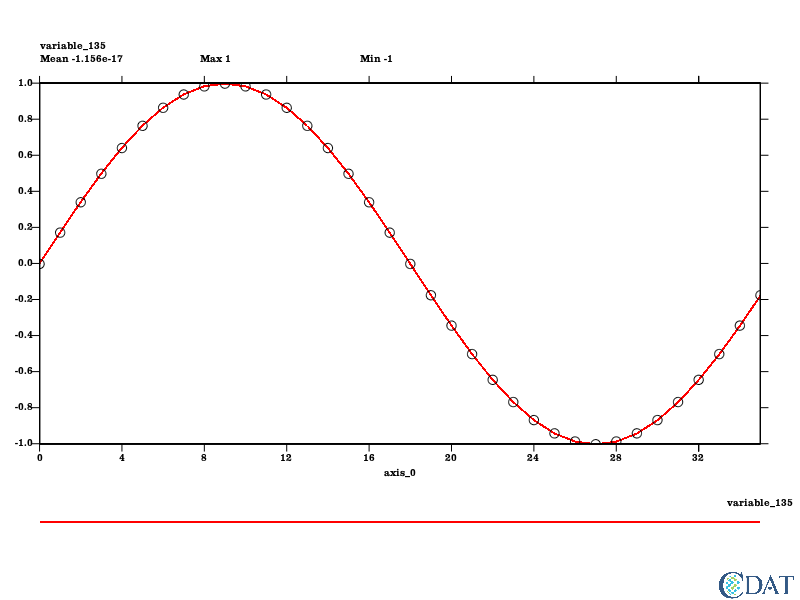## Altering X Axis¶

Back to Top

We would like the axis to be shown in radians, since s is a pure array the x axis is seen by vcs as 0 to 35

Let's create a dictionary to show a few X points

0 -> 0 45degrees = 4.5 in our units = $\pi/4$

In :
angles = {0:'0',9:r'$\pi/2$',18:r'$\pi$', 27:r'$3\pi/2$',36:r'$2\pi$'}
line.xticlabels1 = angles
x.clear()
x.plot(s,line)

Out: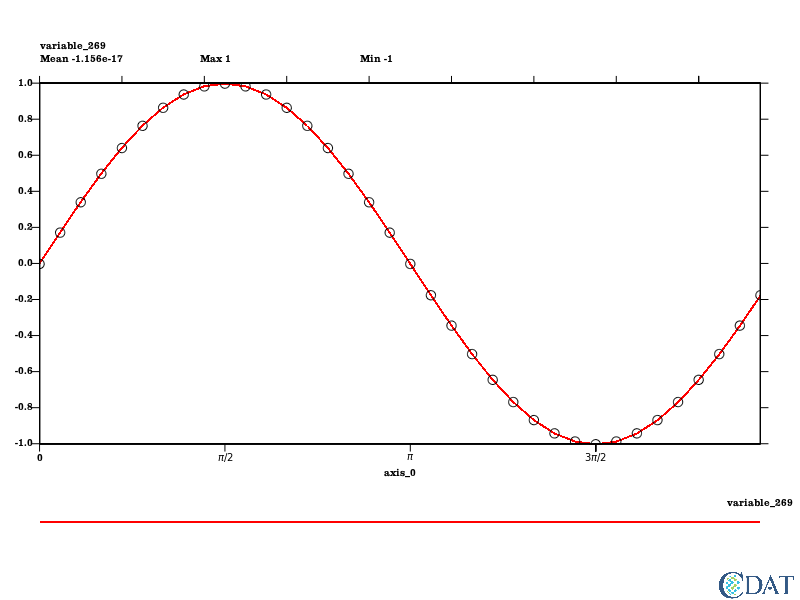But we would like to have the axis labeled "angle" rather than axis_0 so we need to make s a transient variable

In :
import MV2
s = MV2.array(s)
s.id = "sine"
xaxis = s.getAxis(0)
xaxis.id = "angle"
xaxis.units="radians"
# let's make them radians as well
xaxis[:] = xaxis[:]/18.*numpy.pi
# That means we need to match or symbols to new values
angles = {0:'0',numpy.pi/2.:r'$\pi/2$',numpy.pi:r'$\pi$', 3*numpy.pi/2.:r'$3\pi/2$',2*numpy.pi:r'$2\pi$'}
line.xticlabels1 = angles
x.clear()
x.plot(s,line)

Out: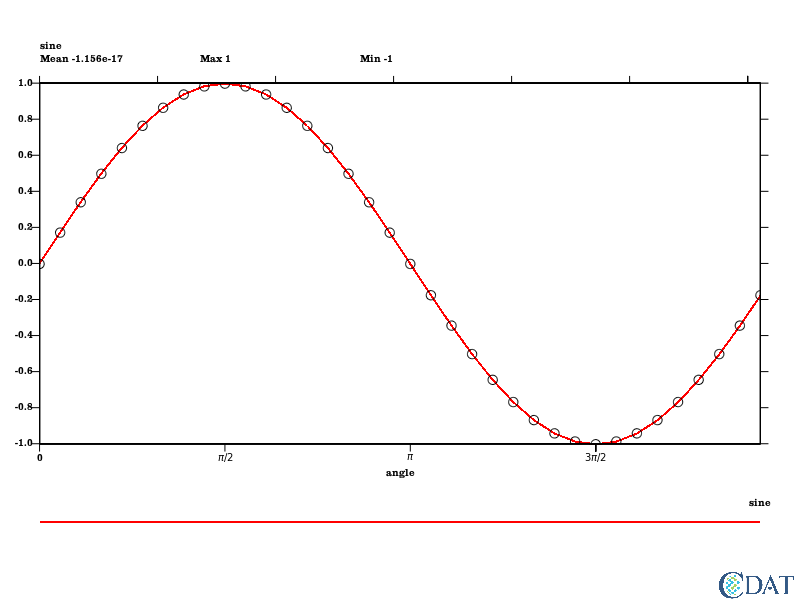The last point being exactly 2$pi$ it is not drawn let's extend our x axis a bit Also by default it is using the first and last value to determine the x values, this can be an issue when plotting multiple line (with different x ref for example).

We recommend setting the x range manually

In :
line.datawc_x1 = -0.1
line.datawc_x2 = numpy.pi*2.000001
x.clear()
x.plot(s,line)

Out: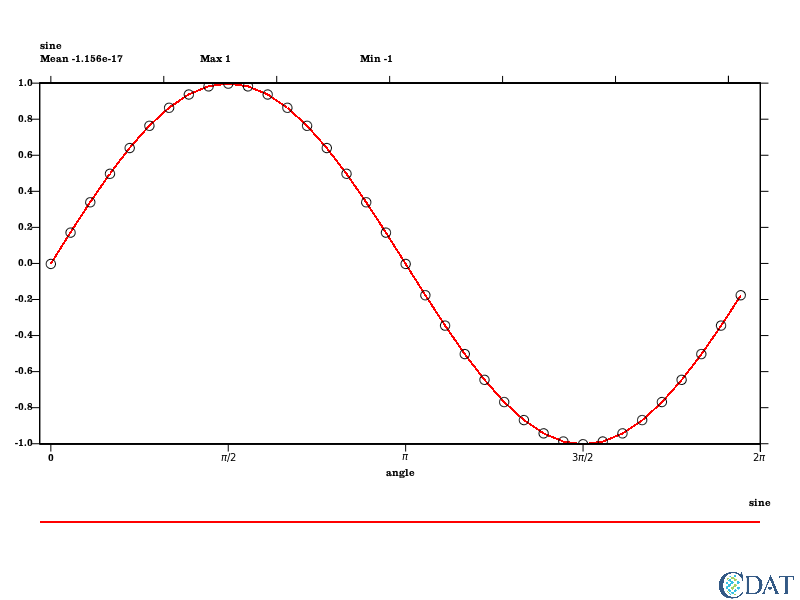## Altering Y Axis¶

Back to Top

We can also tweak the y axis, by default it is using the max and min of the data it is plotting. If you have mutliple line plot this can be an issue as min/max will change with each plot.

We recommend setting the y range manually

In :
line.datawc_y1 = -1.1
line.datawc_y2 = 1.1
line.yticlabels1 = {-1.:"-1",-.5:"-0.5",0:"0",.5:"0.5",1:"1"}
x.clear()
x.plot(s,line)

Out: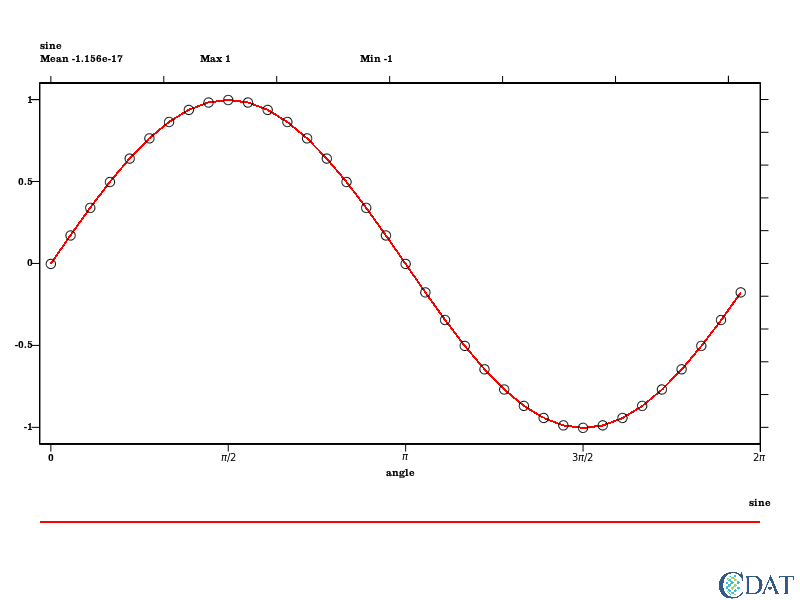## Beautifying the plot some more via templates¶

Back to Top

Let's draw thick line at tickmarks and dotted ones in between

In :
tmpl = vcs.createtemplate()

In :
thick = vcs.createline()  # for thick ticks
thick.width = 1.5  # A bit thicker
thick.color = [[56,56,56]]
dots = vcs.createline()  # for thin/sub ticks
dots.type = ["dot"]
dots.color = [[78,78,78]]
tmpl.xtic1.y2 = tmpl.data.y2  # Ticks extends all the way accross the data section
tmpl.xtic1.line = thick
tmpl.xmintic1.y2 = tmpl.data.y2  # Ticks extends all the way accross the data section
tmpl.xmintic1.priority = 1
tmpl.xmintic1.line = dots
tmpl.ytic1.x2 = tmpl.data.x2  # Ticks extends all the way accross the data section
tmpl.ytic1.line = thick
tmpl.ymintic1.x2 = tmpl.data.x2  # Ticks extends all the way accross the data section
tmpl.ymintic1.priority = 1
tmpl.ymintic1.line = dots
line.xmtics1 = {numpy.pi/4.:"",3*numpy.pi/4:"",5*numpy.pi/4:"",7*numpy.pi/4:""}
line.ymtics1 = {-.75:"", -.25:"", .25:"", .75:""}
x.clear()
x.plot(s,line,tmpl)

Out: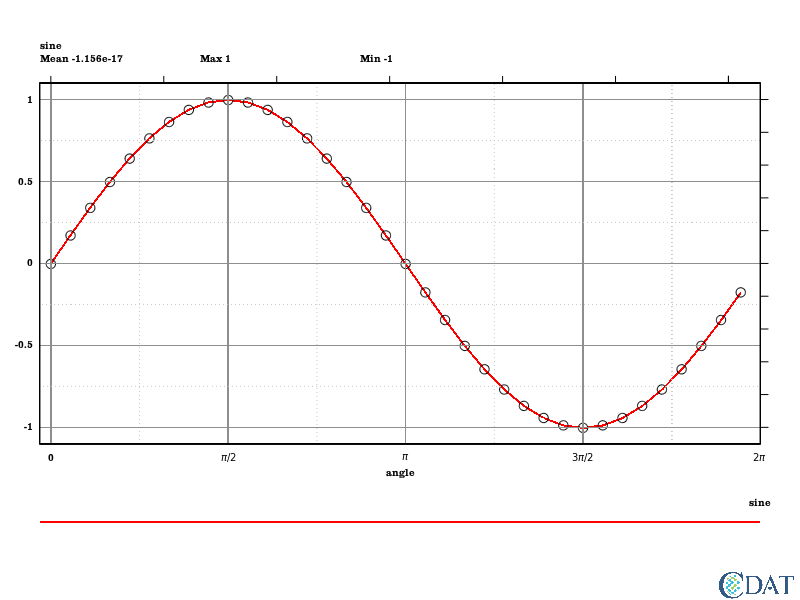In :
black = vcs.createline()
black.width = 2.
tmpl.ymintic2.line = black
tmpl.ymintic2.x1 = tmpl.data.x1
tmpl.ymintic2.x2 = tmpl.data.x2
tmpl.ymintic2.priority = 2  # To be sure it's on top
tmpl.xmintic2.line = black
tmpl.xmintic2.y1 = tmpl.data.y1
tmpl.xmintic2.y2 = tmpl.data.y2
tmpl.xmintic2.priority = 2  # To be sure it's on top
line.ymtics2 = {0:""}
line.xmtics2 = {0:""}
tmpl.box1.line = black  # make outer box match thick line
x.clear()
x.plot(s, tmpl, line)

Out: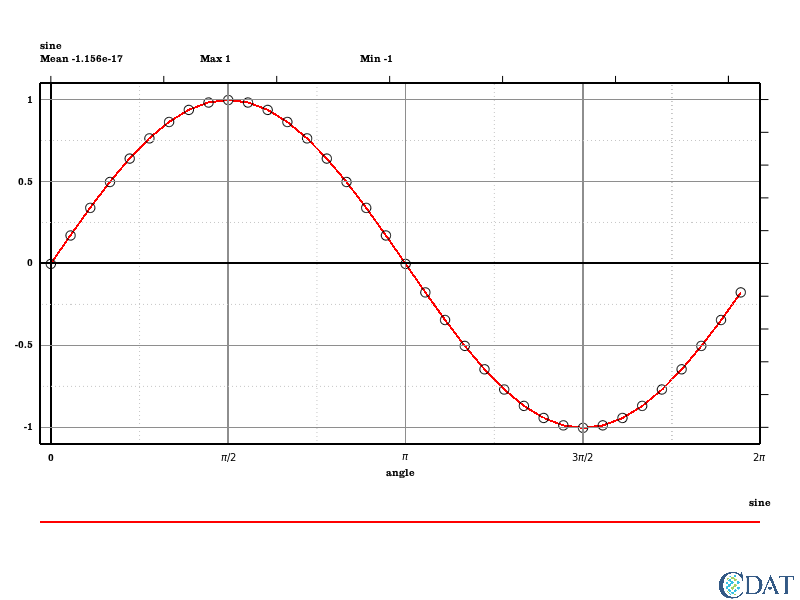## Multiple Lines¶

Back to Top

Let's plot multiple lines

In :
c = MV2.cos(angle/180.*numpy.pi)
c.id = "cosine"
c.setAxis(0,xaxis)

x.clear()
x.plot(s,tmpl,line)
x.plot(c,line,tmpl)  # order does not matter

Out: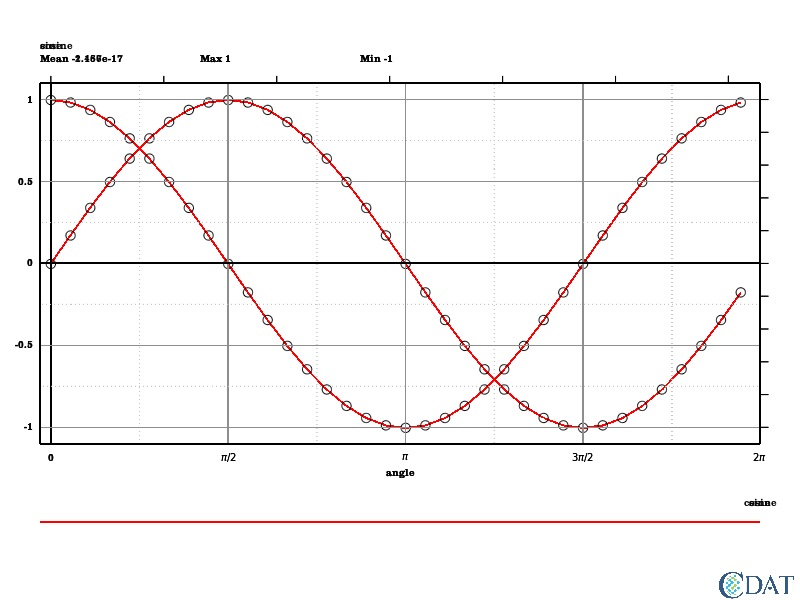We are almost there but we need to blank a few attributes on template (name, min, max, etc.. and legend) Also we need a different color for the cosine

In :
tmpl.blank(["dataname","min","mean","max"])
line_cos = vcs.create1d(source=line)
line_cos.linecolor = "blue"
line_cos.marker = "diamond"
line_cos.markersize = 1.5
line_cos.markercolor = "black"
x.clear()
x.plot(s,tmpl,line)
x.plot(c,tmpl,line_cos)

Out: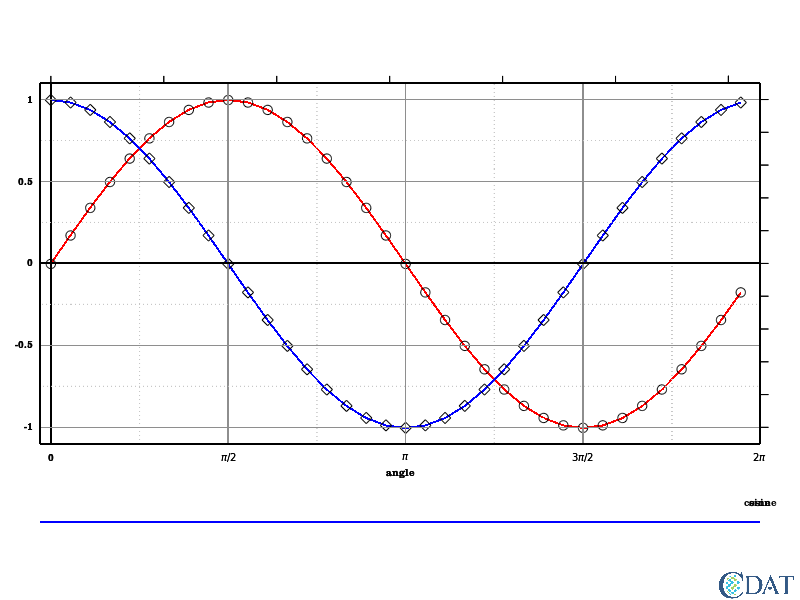Ok now we still have to tweak the legend, templates have a built in function for this

In :
# First let's blank the legend default
x.clear()
tmpl.legend.priority=1  # Make sure it is on
tmpl.drawLinesAndMarkersLegend(x, [line.linecolor, line_cos.linecolor],
[line.linetype, line_cos.linetype],
[line.linewidth,line_cos.linewidth],
[line.markercolor, line_cos.markercolor],
[line.marker, line_cos.marker],
[line.markersize, line_cos.markersize],
[s.id, c.id])
tmpl.legend.priority=0
x.plot(s,tmpl,line)
x.plot(c,tmpl,line_cos)

Out: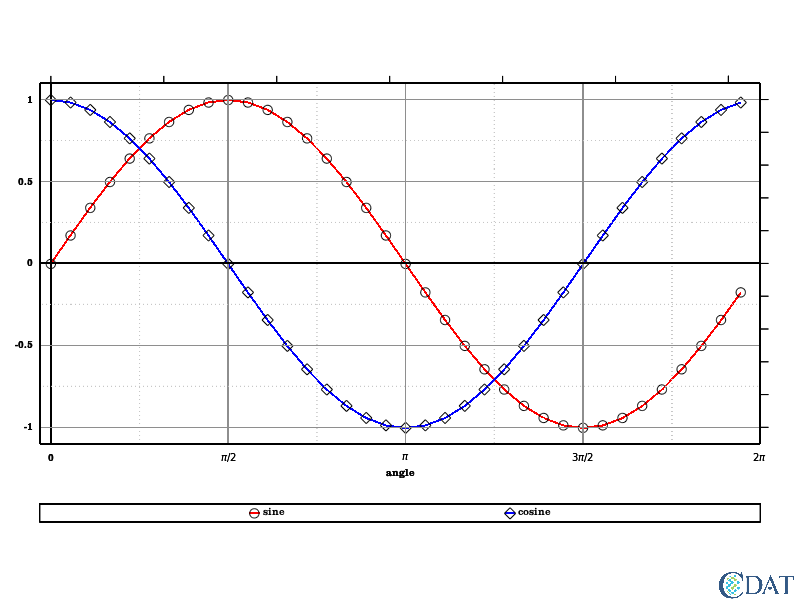And finally let's use to template to beautify a bit more

In :
x2 = .7
tmpl.data.x2 = x2
# We also need to move the other things linked to it
tmpl.box1.x2= x2
tmpl.ymintic1.x2 = x2
tmpl.ymintic2.x2 = x2
tmpl.ytic1.x2 = x2
tmpl.ytic2.x1 = x2
tmpl.ytic2.x2 = x2+.01

# Let's move the legend
tmpl.legend.x1 = x2+.05
tmpl.legend.x2 = .9
tmpl.legend.y1 = .45
tmpl.legend.y2 = .55

x.clear()
tmpl.legend.priority=1  # Make sure it is on
# Let's stack it vertically now
tmpl.drawLinesAndMarkersLegend(x, [line.linecolor, line_cos.linecolor],
[line.linetype, line_cos.linetype],
[line.linewidth,line_cos.linewidth],
[line.markercolor, line_cos.markercolor],
[line.marker, line_cos.marker],
[line.markersize, line_cos.markersize],
[s.id, c.id],
stacking="vertical")
tmpl.legend.priority=0
x.plot(s,tmpl,line)
x.plot(c,tmpl,line_cos)

Out: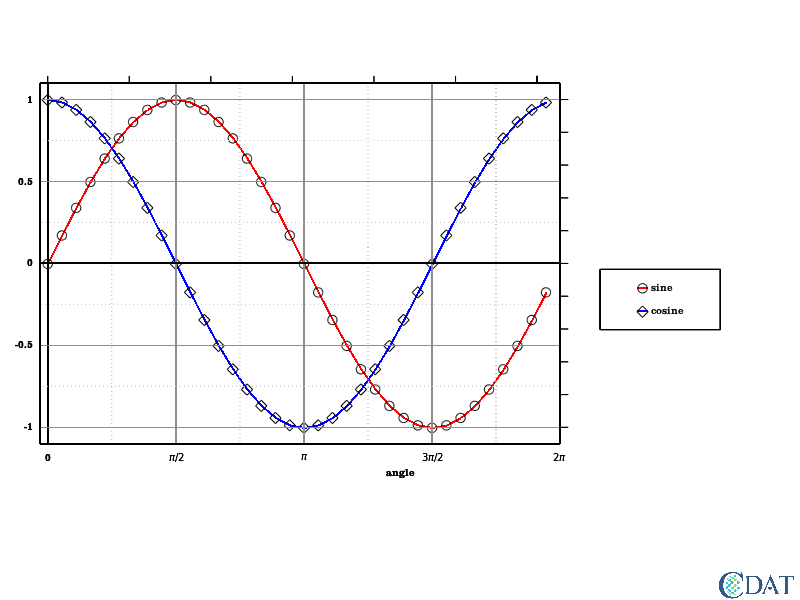## Other types of 1D plots¶

Back to Top

### Flipping axes (aka x(y) vs y or xyvsy)¶

Back to Top

In :
line_flip = vcs.create1d(source=line)
line_flip.flip = True
# Note that f = vcs.createxyvsy() creates a 1d plot with flip = True
def flip(gm, attr):
tmp = getattr(gm, "x"+attr)
setattr(gm, "x"+attr, getattr(gm,"y"+attr))
setattr(gm, "y"+attr, tmp)

for att in ["mtics","ticlabels"]:
for number in ["1", "2"]:
flip(line_flip, att + number)

line_flip.datawc_x1 = -1.1
line_flip.datawc_x2 = 1.1
line_flip.datawc_y1 = -.1
line_flip.datawc_y2 = numpy.pi*2.1
x.clear()
x.plot(s,line_flip, tmpl)


Out: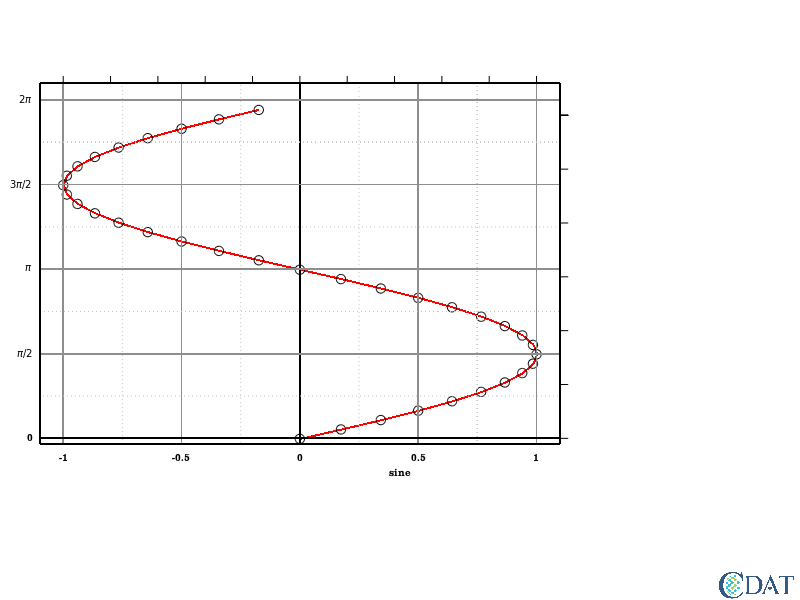### Scatter plots aka y vs x¶

Back to Top

Let's plot cosine vs sine

In :
scatter = vcs.create1d(source=line)
scatter.datawc_x1 = -2.2
scatter.datawc_x2 = 2.2
scatter.xmtics1 = scatter.ymtics1
scatter.xmtics2 = scatter.ymtics2
scatter.xticlabels1 = scatter.yticlabels1
scatter.xticlabels2 = scatter.yticlabels2
x.clear()
# x values passed first
x.plot(2*s,c,scatter)

Out: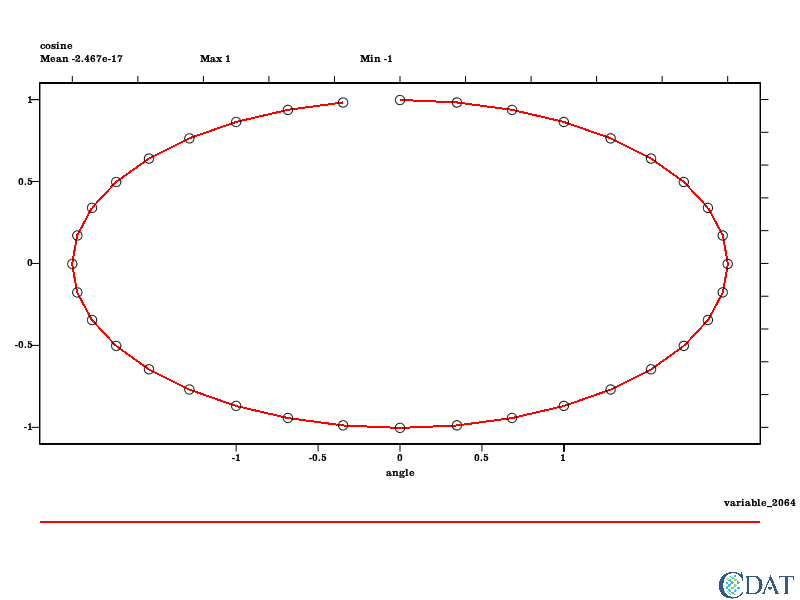Let's turn off the lines

In :
scatter.linecolor= [0,0,0,0]  # no opacity = transparent
x.clear()
# x values passed first
x.plot(2*s,c,scatter)

Out: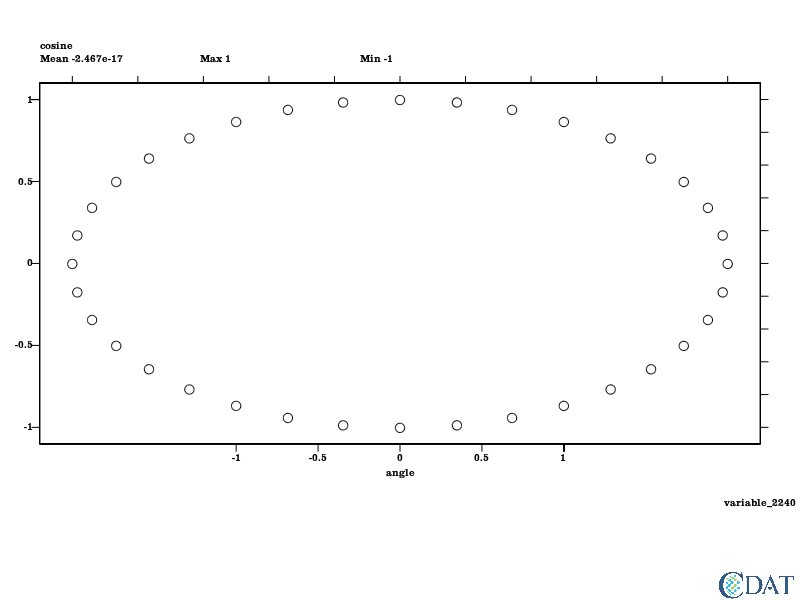Or turn off the markers

In :
scatter.linecolor = "red"
scatter.markercolor = [0,0,0,0]
x.clear()
# x values passed first
x.plot(2*s,c,scatter)

Out: